### CATALOG NO. B38 2010EightEight fully open

Specifications Edition of 8
8" diameter, digital reproduced ink drawings, cast acrylic covers

Collections
MIT Rotch Library
Evergreen State College
University of Michigan Fine Arts Library
University of Pittsburgh
Seattle University
University of Puget Sound
UCLA Arts Library Artist Book Collection

### Also see Eight - Unique book

﻿ Eight is a visual experiment using eight different mathematical operations on the same set of eight random numbers to produce a framework of arcs and circles for eight sets of abstract drawings.

The mathematical operations are as follows: sum, difference, product, quotient, raising to the 8th power, taking the 8th root, sum series, and product series. The random numbers, as seen on the title page, are: .739, .016, .376, .659, .175, .039, .703, .775

Each set of drawings has eight elements. Each element is composed of a portion of a circle or arc that has its diameter determined by the result of one of the eight mathematical operation performed on each random number, one mathematical operation per page.

The different mathematical operations produce widely varying results as to scale. Some arcs are almost straight lines and some circles have to be represented as dots. The drawing scale varies from 3 to 1 to more than 4,000,000 to 1.

The order of the random number results listed on each page corresponds to the order of the circle or arc elements, top to bottom. The mathematical operation is depicted at the top of the random number result list and the scale of the arc-circle elements is listed at the bottom. The drawings are pure abstractions drawn along the arc or circle formed by the mathematical operation.

“Eight” was originally created as a unique book in 1999. This edition of eight copies was created at two-thirds scale (8” diameter) in 2010.Eight closedTitle page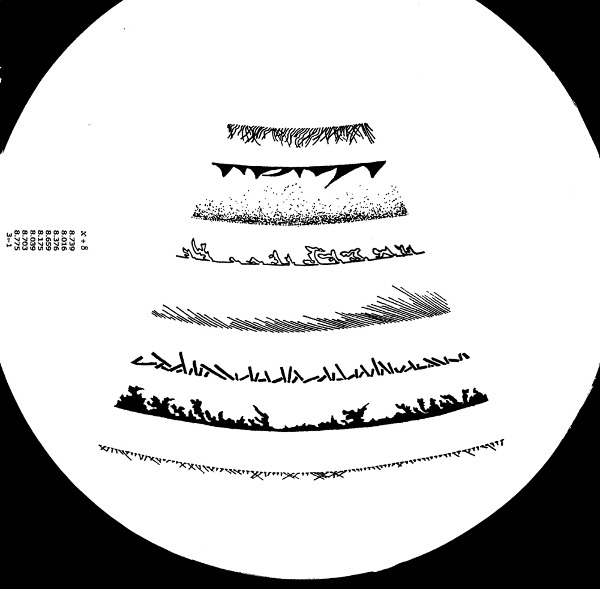Page 1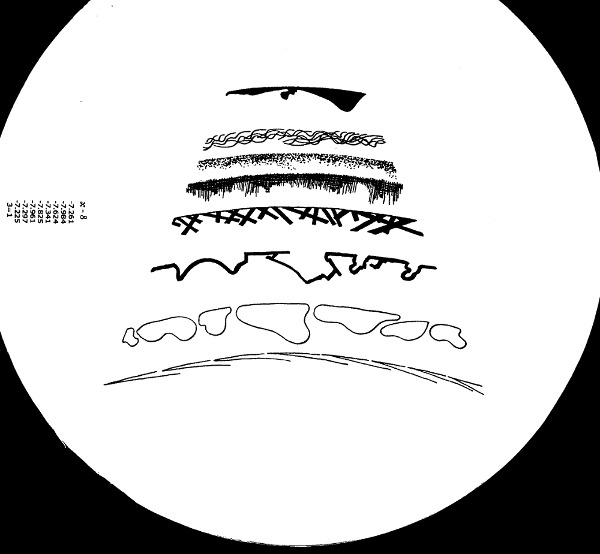Page 2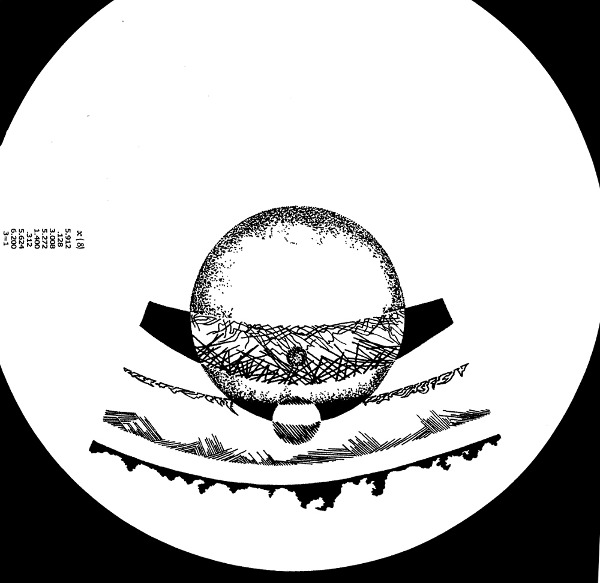Page 3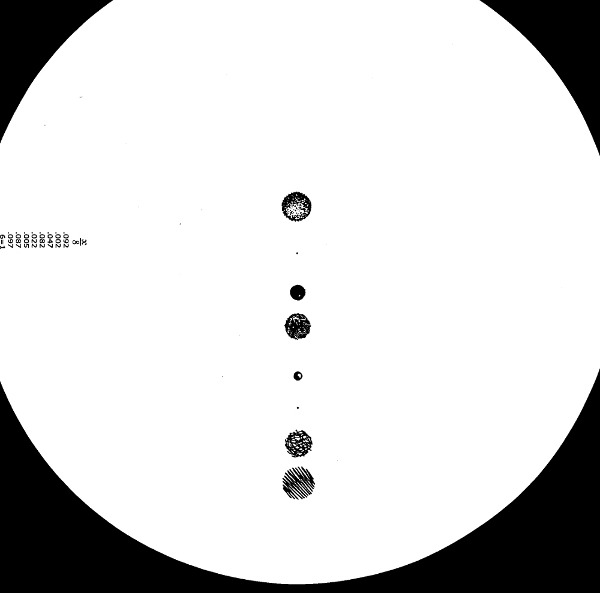Page 4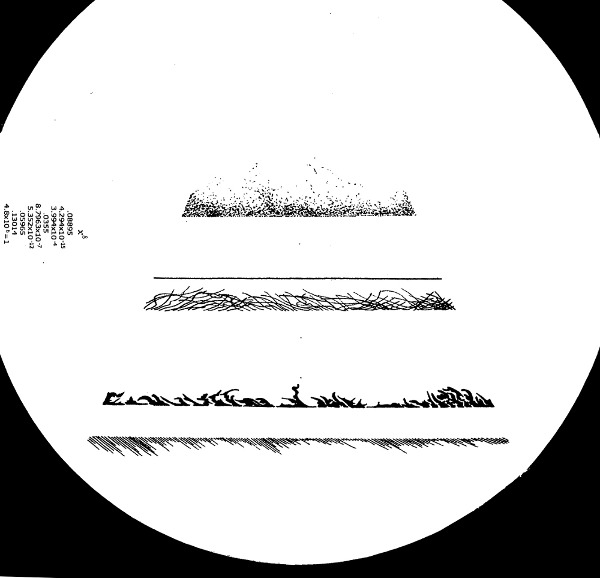Page 5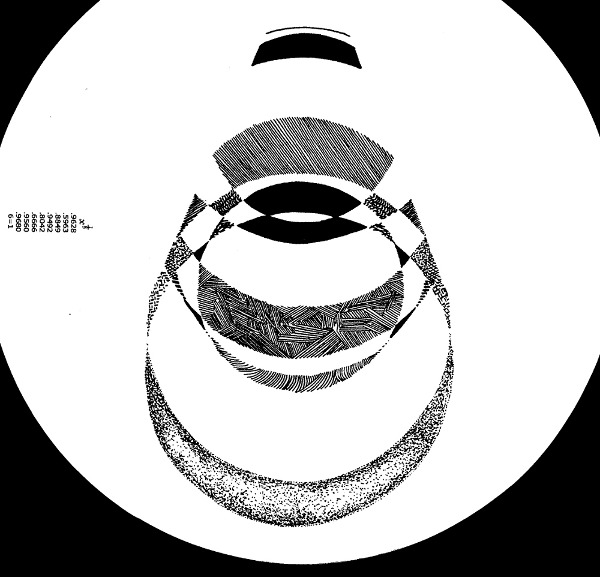Page 6Page 7Page 8